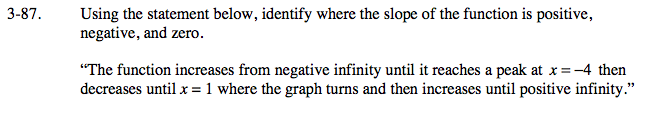### Home > CALC > Chapter Ch3 > Lesson 3.3.1 > Problem3-87

3-87.

Using the statement below, identify where the slope of the function is positive, negative, and zero.
"The function increases from negative infinity until it reaches a peak at x = −4 then decreases until x = 1 where the graph turns and then increases until positive infinity." Homework Help ✎When describing a function, how do the words 'increase' and 'decrease' connect with slope?